Next: Landau Parameters from the Up: Infinite Nuclear Matter Previous: Equation of state'' of

## Pressure, Incompressibility and Asymmetry Coefficients

At the saturation point, all first derivatives of the energy per nucleon have to vanish and all second derivatives have to be positive. The first derivative with respect to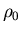is related to the pressure, the second derivative with respect tois related to the incompressibility, and the second derivatives with respect to the Ii is related to the asymmetry coefficients. For symmetric matter, the first derivatives with respect to the Ii vanish because the energy per nucleon is an even function of all Iis. The pressure is given by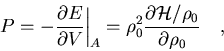(48)

which gives
 P =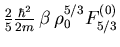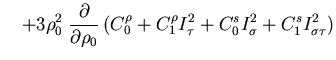(49)

The incompressibility is defined as
(50)

which, for the Skyrme energy functional (49) at the saturation point (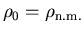,
) gives
=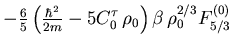(51)

The asymmetry coefficients are:
=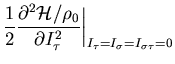=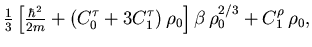(52)
=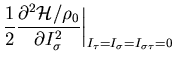=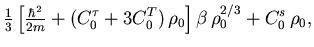(53)
=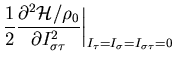=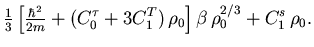(54)

Here,is the well-known volume asymmetry coefficient of the liquid-drop model, andandare its generalizations to the spin and spin-isospin channels of the interaction. At the saturation point, all asymmetry coefficients have to be positive.Next: Landau Parameters from the Up: Infinite Nuclear Matter Previous: Equation of state'' of
Jacek Dobaczewski
2002-03-15